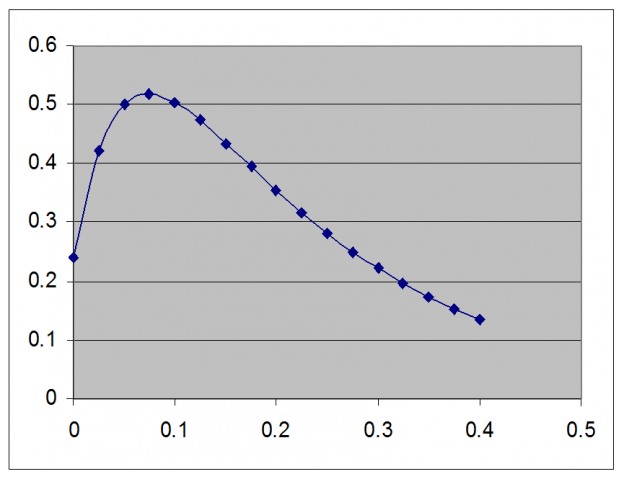# DO (Dissolved Oxygen) and related numericals

May 3 • General • 2446 Views • 2 Comments on DO (Dissolved Oxygen) and related numericals

DO(Dissolved oxygen) content is one of the factor that is taken into consideration when determining the water quality.DO is in equilibrium based on gas pressure and temperature.DO changes with change in these condition.Methods to test DO are as follows-

1.Winkler Titration Method-

• Take water sample and halve it.Keep one sample in dark for BOD analysis.
• Add 2ml aqueous MnCl2(dissolve 4g MnCl2 in 10ml of distilled water) and 2ml alkaline iodine solution(dissolve 3.3g NaOH and 2g KI in 10ml of distilled water) to other sample of water and shake it.
• Now add 2ml concentrated HCl to sample and shake it well.The iodine formed is directly proportional to the DO.
• Titration is done by taking 50ml of above solution and 0.0125M sodium thiosulfate solution.Here,starch is used as indicator.When the blue-black color disappear then the end point is reached.

2.Colorimetric Method-

• In this method two water samples are collected,one for DO test and other for BOD test.The sample must be collected under water to ensure that there is no  trapped air bubbles.For this method a field kit is available using “Smart” colorimeter.

Q1.20ml of river water is collected from just below the brewery,2ml of river water diluted to 1L,aerated and seeded.Initially,the DO content was 7.8mg/L.After 5days,the DO content had dropped to 5.9mg/L and after 20 days it dropped to .3mg/L.What is the ultimate BOD?

Ans.We know,

BODE = BOD (1-e-kt),

where,k is a rate constant

BOD is a constant.

here,we have 4 unknowns.If we know 2 of them for example BODE at a given time,even then we are left with rest 2 unknowns.To determine 2 unknowns we require 2 equations.

BOD5 = BOD (1-e-k(5 days))

BOD5 = 7.8 mg/L – 5.9 mg/L / 2 mL/1000 mL = 950 mg/L

BOD20 = BOD (1-e-k(20 days))

BOD20 = 7.8 mg/L – 5.3 mg/L / 2 mL/1000 mL = 1250 mg/L

Now by taking the ratio of above equations,we get

BOD5 / BOD20 = BOD (1-e-k(5 days)) / BOD (1-e-k(20 days))

BOD5 / BOD20=(1-e-k(5 days)) / (1-e-k(20 days))

The value of BOD5 and BOD20 is given in the problem.Let the ratio,BOD5/BOD20 be Q.

Q=BOD5 / BOD20=950 /  1250=0.76

0.76 = (1-e-k(5 days)) / (1-e-k(20 days))

Now,we have to solve for k.

0.76(1- e-k(20 days)) = (1-e-k(5 days))

0.76 – 0.76 e-k(20 days)) = 1-e-k(5 days)

e-k(5 days) – 0.76 e-k(20 days) = 1 – 0.76=0.24

Now plotting graph-k=0.28 day-1

the ultimate BOD,BOD5 = BOD (1-e-k(5 days))

950 mg/L = BOD (1 – e-(0.28)(5))

BOD = 1261 mg/L

Now let us assume k=0.23 days-1

BOD5 = BOD (1-e-k(5 days))

950 mg/L = BOD (1 – e-(0.23)(5))

BOD = 1390 mg/L

Again calculating BOD from the 20 days data,we get

BOD20 = BOD (1-e-k(20 days))

1250 mg/L = BOD (1 – e-(0.23)(20))

BOD = 1262 mg/L

The ultimate BOD will not agree since the k value is wrong.Since we re averaging more days,the reaction should be 99% complete if the assumed k is even partially correct.Hence,20 days should always be more accurate.

### 2 Responses to DO (Dissolved Oxygen) and related numericals

1. priyanka nayak says:

These days the amount of dissolved oxygen in the water bodies keeps on decreasing due to environmental changes. It is very important for us to keep the level of dissolved oxygen in a balanced form.

2. vishrut patel says:

Numerical based on DO(dissolved oxygen) is well described which help us to know about the water quality …